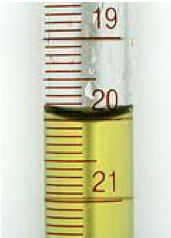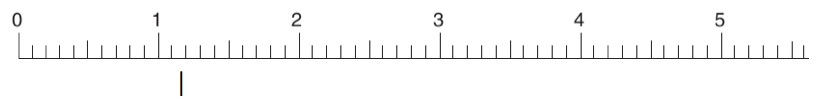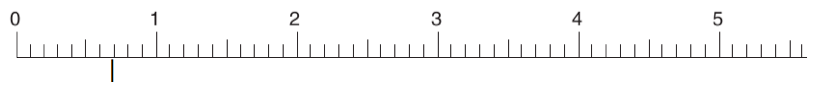## Analytical Chemistry

Learn the toughest concepts covered in your Analytical Chemistry class with step-by-step video tutorials and practice problems.

3. Experimental Error

# Significant Figures

Significant figures are necessary to communicate the level of accuracy with which values are recorded.

Significant Figures
1
concept

## Significant Figures2m
Play a video:
So here we're going to say that significant figures indicate the level of precision involved with measurements and recordings and when we get to ideas such as uncertainty, we'll see significant figures playing a bigger role. Now we're gonna say a number with more significant figures is more precise. Now we're gonna say determine the number of significant figures for any given value can be easy depending on how you do it. There's a lot of rules associated with significant figures. But to make it simpler for ourselves we'll break it down into two simple ideas and it has to do with the presence of a decimal point or not. So if we take a look here, we're gonna say for significant figures are first rule is this if your number has a decimal point you're gonna move from left to write removed from left to right. You're gonna start counting once you get to your first non zero number and keep counting until the end. So our first non zero number as we're moving from left to right, is this too? So that's where we start counting and we count all the way until the end. So 123. This would have three significant figures. Now if your number doesn't have a decimal point they're gonna move from right to left. We're gonna start counting again. Once we get to our first non zero number and keep counting into the end. So our first number here is five. So keep counting all the way into the end. So 1234, this would have four significant figures. And to keep significant figures easy for us to understand we're gonna go by these two simple rules. Now there'll be other things that pop up, which we'll talk about in the following example. But for right now, just realize that we have these two basic rules to help us with significant figures. Now that we've done that. Let's move on to our example.
2
example

## Significant Figures2m
Play a video:
So here we're gonna take a look at this example here it says, how many Sig Figs does each number contain? Alright, so for the first one we're starting out with 10.0 10 10 m because it possesses a decimal point we're gonna move from left to right. And we're gonna start counting once we get to our first non zero number. So here's our first non zero number. Once you start counting you count all the way until the end. So this would be 1234 Sig Figs for this particular value. For the next one We have 10,030 seconds it has no decimal point that we can see. So we're gonna move from right to left again. Start counting once you get your first non zero number which in this case would be the three and count all the way into the end. So 1234. So this would also have four sig figs. Next we have 2.00 times 10 to the three leaders. We don't worry about the base and our exponent, all we care about is the coefficient because this is written in scientific notation because it has a decimal, we're gonna go from left to right. Our first non zero number is this too? And we count all the way into the end. So 12366. Sing things. Now. Finally we have 14 people. Now this is what we call an exact number because you're not gonna have 14.1 or 14.30 people you're gonna have a whole number of people, an exact number of people. When we're dealing with exact numbers, there is actually an infinite number of significant figures. Now the chances of you seeing this, it all depends on your professor. But just remember when it comes to Sig Figs, we have two easy rules that we even remember in terms of decimal point or no decimal point. But when we're dealing with an exact number, there's an infinite ob series of sick fix. Because 14 people could be written as 14 people or 14.0 people or 14.0 people, all of them are saying the same thing. And because of that, there's an infinite number of sig fix. So just remember when we're dealing with uh an exact number, there's an infinite number of significant figures involved.

● Multiplication/Division: Measurement with least SIG FIGS determines final answer.

3
example

## Significant Figures3m
Play a video:
4
example

## Significant Figures1m
Play a video:5
Problem

Read the ruler measurement to the correct number of significant figures.6
Problem

Read the ruler measurement to the correct number of significant figures.